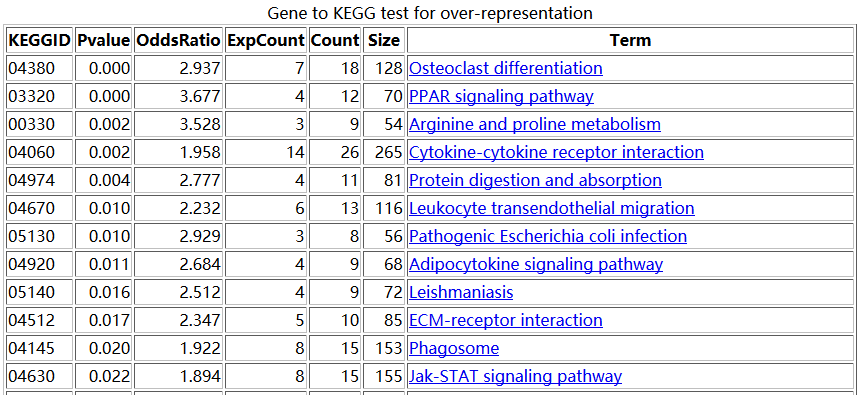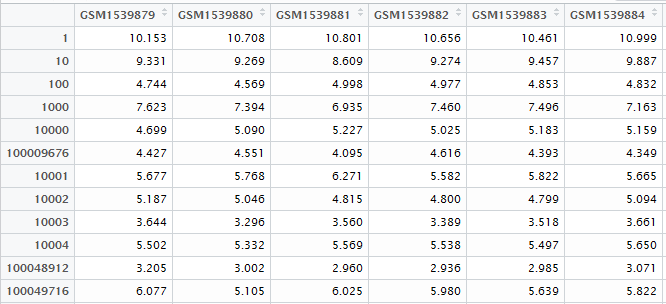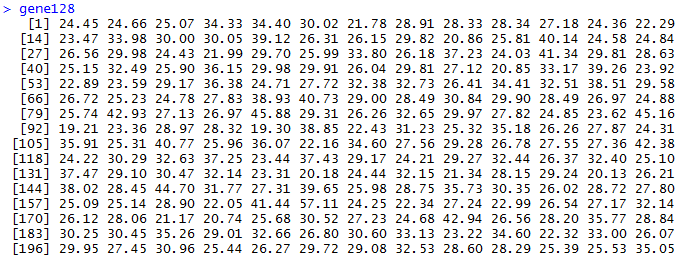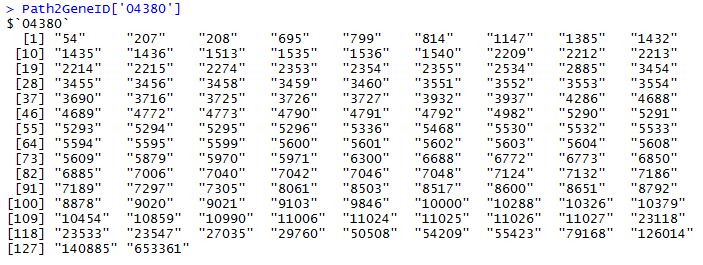# 用重抽样+主成分方法来做富集分析setwd("D:\\my_tutorial\\补\\用limma包对芯片数据做差异分析")

rownames(exprSet)=exprSet[,1]

exprSet=exprSet[,-1]

exprSet=exprSet[,-c(1:2)]

library(hgu133plus2.db)

library(annotate)

platformDB="hgu133plus2.db";

probeset <- rownames(exprSet)

rowMeans <- rowMeans(exprSet)

EGID <- as.numeric(lookUp(probeset, platformDB, "ENTREZID"))

match_row=aggregate(rowMeans,by=list(EGID),max)

colnames(match_row)=c("EGID","rowMeans")

dat=data.frame(EGID,rowMeans,probeset)

tmp_prob=merge(dat,match_row,by=c("EGID","rowMeans"))

relevantProbesets=as.character(tmp_prob\$probeset)

length(relevantProbesets) #hgu133plus2.db  20156

exprSet=exprSet[relevantProbesets,]

EGID_name=as.numeric(lookUp(relevantProbesets, platformDB, "ENTREZID"))

rownames(exprSet)=as.character(EGID_name)

d=exprSetgene128=sapply(1:1000,function(y) {

dat=t(d[sample(row.names(d), 128, replace=TRUE), ]);

round(100*summary(fast.prcomp(dat))\$importance[2,1],2)

}

)

>  summary(gene128)

Min. 1st Qu.  Median    Mean 3rd Qu.    Max.

19.1    25.8    28.8    29.8    32.5    59.7path_04380_gene=intersect(rownames(d),as.character(Path2GeneID[['04380']]))

dat=t(d[path_04380_gene,]);

round(100*summary(fast.prcomp(dat))\$importance[2,1],2)1-pnorm((38.83-mean(gene128))/sd(gene128))

 0.0625